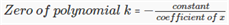# Zeros Of polynomial

For a polynomial, there could be some values of the variable for which the polynomial will be zero. These values are called zeros of polynomial. General form of a polynomial in x is anxn + an-1xn-1 +….. + a1x + a0, where an, an-1, ….. , a1, a0 are constants, a≠0 and n is a whole number. For example, algebraic expressions such as √x + x + 5, x2 + 1/x2 are not polynomials because all exponents of x in terms of the expressions are not whole numbers.

## Finding Zeros of Polynomials

Zeros of a polynomial can be defined as the points where the polynomial becomes zero on the whole. A polynomial having value zero (0) is called zero polynomial. Degree of a polynomial is the highest power of the variable x.

• Polynomial of degree 1 is known as linear polynomial.
Standard form is ax + b, where a and b are real numbers and a≠0.
2x + 3 is a linear polynomial.
• Polynomial of degree 2 is known as quadratic polynomial.
Standard form is ax2 + bx + c, where a, b and c are real numbers and a ≠ 0
x2+ 3x + 4 is an example for quadratic polynomial.
• Polynomial of degree 3 is known as a cubic polynomial.
Standard form is ax3+ bxx2 + cx + d, where a, b, c and d are real numbers and a≠0.
x3 + 4x + 2 is an example for cubic polynomial.

Similarly,

y6 + 3y4 + y is a polynomial in y of degree 6.

### Examples

Example: What is the value of a if degree of polynomial x3 + xa-4 + x2 + 1 is 4?

Degree of a polynomial P(x) is the highest power of x in P(x).

Therefore, xa-4  = x4

a-4 = 4, a = 4+4 =8

Consider, P(x)= x2 – 3x + 2,

Put x = 3 in P(x) which gives,

P(3) = 9 – 9 + 2 = 2

Replace x by 2 in the polynomial x2 – 3x + 2, which gives P(2) = 4 – 6 + 2 = 0

Similarly, value of x2 – 3x + 2, at x = 0 is,

P(0) = 0-0+2 = 2

In general; if P(x) is a polynomial in x and k is any real number, then value of P(k) at x = k is denoted by P(k) is found by replacing x by k in P(x).

In the polynomial x2 – 3x + 2,

Replacing x by 1 gives,

P(1) = 1 – 3 + 2 = 0

Similarly, replacing x by 2 gives,

P(2) = 4-6+2 = 0

For a polynomial P(x), real number k is said to be zero of polynomial P(x), if P(k) = 0.

Therefore, 1 and 2 are the zeros of polynomial x2 – 3x + 2.

## Zeros of Polynomial Formula

Consider, P(x) = 4x + 5 to be a linear polynomial in one variable.

Let a be zero of P(x), then,

P(a) = 4k+5 = 0

Therefore, k = -5/4

In general, If k is zero of the linear polynomial in one variable; P(x) = ax +b, then

P(k) = ak+b = 0

k = -b/a

It can also be written as,We have discussed polynomials and zeros of a polynomial here. To know more about polynomials, download Byjus-the learning app.